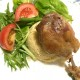# Ideal Math EducationMath Online Tom Circle\$latex boxed { text {Ideal Math Education } = (C + E) * F}&fg=aa0000&s=3\$

C = Chinese 中文 = Primary school Arithematics sans “Algebra” (= Singapore Modeling Math). Abacus-Algorithmic thinking.

E = English = Secondary School Math
=> Applied, Tricky, Math Olympiad-style
=> Engineering, Business, Applied Science.

F = Français (French) = High-school / Baccalaureate & University Math = Theoretical, Abstract
=> Rigorous Math Philosophy for Advanced Concepts
=> New frontier Scientific Research.

Why (C+E) ?
C + E = Basic Math Foundation.

Why * F ?
If multiply by Theoretical F, like flying with added wings (如虎添翅)。

However,
if (C+E) -> 0 (less applied), or
if F -> 0 (lack theories),
then Total Math Education -> 0.

View original post## Author: tomcircle

Math amateur

Posted on Categories math

This site uses Akismet to reduce spam. Learn how your comment data is processed.